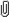October 05, 2023, 01:05:59 am

###AuthorTopic: Mathematics Extension 1 Challenge Marathon  (Read 25276 times) Tweet Share

0 Members and 1 Guest are viewing this topic.

#### Opengangs

• New South Welsh
••• Posts: 718
• $\mathbb{O}_\mathbb{G}$
• Respect: +480##### Re: Mathematics Extension 1 Challenge Marathon
« Reply #45 on: December 29, 2018, 02:14:35 am »
+1
$a) \text{ Show that } \\ (a + b + c)^2 = a^2 + b^2 + c^2 + 2(ab + ac + bc)$
$\text{Let } p(x) = x^3 + 12x^2 - 2x + 1 \text{ have roots } \alpha, \beta, \gamma. \\ b) \text{ Explain why} \\ \alpha^3 = 2\alpha - 12\alpha^2 - 1.$$c) \text{ Hence, or otherwise, find } \alpha^3 + \beta^3 + \gamma^3.$

#### david.wang28

• MOTM: MAR 19
• Forum Obsessive
•• Posts: 223
• Always do everything equanimously
• Respect: +29##### Re: Mathematics Extension 1 Challenge Marathon
« Reply #46 on: December 30, 2018, 11:41:07 pm »
+1
$a) \text{ Show that } \\ (a + b + c)^2 = a^2 + b^2 + c^2 + 2(ab + ac + bc)$
$\text{Let } p(x) = x^3 + 12x^2 - 2x + 1 \text{ have roots } \alpha, \beta, \gamma. \\ b) \text{ Explain why} \\ \alpha^3 = 2\alpha - 12\alpha^2 - 1.$$c) \text{ Hence, or otherwise, find } \alpha^3 + \beta^3 + \gamma^3.$
Selection rank: 95.25

2020-2024: Bachelor of engineering and computer science @ UNSW

#### Opengangs

• New South Welsh
••• Posts: 718
• $\mathbb{O}_\mathbb{G}$
• Respect: +480##### Re: Mathematics Extension 1 Challenge Marathon
« Reply #47 on: February 05, 2019, 02:45:56 pm »
+1
Not so much of a "challenge" but an interesting proof to a property you guys should be familiar with.
« Last Edit: February 05, 2019, 02:50:00 pm by Opengangs »

#### Opengangs

• New South Welsh
••• Posts: 718
• $\mathbb{O}_\mathbb{G}$
• Respect: +480##### Re: Mathematics Extension 1 Challenge Marathon
« Reply #48 on: May 14, 2020, 10:55:24 pm »
+1
A fun (and not too hard) problem that ties into number theory a little :-))

Our goal here is to investigate $n^5 - n$ and show that, indeed it is divisible by 5. We will verify this is true using induction (that's for you to prove) at the end. But for now, let's explore this using some number theory.

Part 1: Show that $n^5 - n$ can be written as $n(n - 1)(n + 1)(n^2 + 1)$.
Part 2: If we choose $n = 5k$, $n = 5k + 1$ or $n = 5k + 4$, briefly explain why $n^5 - n$ is divisible by 5 for any integer $k$.
Part 3: Show that, if we choose $n = 5k + 2$ or $n = 5k + 3$, then $n^5 - n$ is also divisible by 5.
Part 4: Conclude that, regardless of our choice of $n$, $n^5 - n$ is always divisible by 5.
Part 5: Verify this statement using induction. That is, prove that $n^5 - n$ is divisible by 5 using mathematical induction.